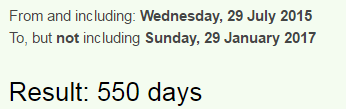## Cipher## Jan 29, 2017

### People drifting in waters off Malaysian coast await rescue

CNN's post before any updates -  http://edition.cnn.com/2017/01/29/asia/malaysia-boat-missing-tourists/index.htmlPeople drifting in waters off Malaysian coast await rescue = 228 (Reduced)
Death = 24+30+6+120+48 = 228 (Sumerian)44th prime number is 193 (Kill = 44, Execution = 44)This story reminds me of the Malaysian story which is entirely coded out with crazy numbers, Malaysian airplane disappeared on 3/8/2014 with 227 passengers
227 is one of the special numbers, the airplane that disappeared was Boeing 777, 227 converted to Octal is 343 (7x7x7), it is also the number connected to Pi - 22/7 = 3.14 and both 227 and 314 are very frequently coded
The incident occurred during the Chinese New Year celebration period.The boat sank off coast of Sabah in Malaysia
coast of Sabah = 3+6+1+1+2 + 6+6 + 1+1+2+1+8 = 38 (Reduced)
Death = 4+5+1+20+8 = 38 (Ordinal)

Sabah = 1+1+2+1+8 = 13 (Reduced)
Sabah = 19+1+2+1+8 = 31 (Ordinal)
31 people were on the boat and the police report was lodged 13 hours later
The story comes on a date with 67 numerology (1+29+20+17 = 67)
Three hundred thirty one = 100+8+80+5+5 + 8+200+40+4+80+5+4 + 100+8+9+80+100+400 + 50+40+5 = 1331 (Jewish)
They were traveling on a catamaran
catamaran = 3+1+2+1+4+1+9+1+5 = 27 (Reduced)
Malaysia = 4+1+3+1+7+1+9+1 = 27 (Reduced)

Malaysian coast = 13+1+12+1+25+19+9+1+14 + 3+15+1+19+20 = 153 (Ordinal)
twenty eight Chinese tourists = 2+5+5+5+2+7 + 5+9+7+8+2 + 3+8+9+5+5+10+5 + 2+6+3+9+9+10+2+10 = 153 (S Exception)
28th prime number is 107 (107 converted to Octal is 153, 17th triangular number is 153)

The boat is believed to have sunk near Mengalum Island after it sailed from Kota Kinabalu, and two crew members managed to make their way to land to seek assistance, the report says.
Mengalum Island = 4+5+5+7+1+3+3+4 + 9+1+3+1+5+4 = 55 (Reduced)
Kota Kinabalu = 11+6+2+1 + 11+9+5+1+2+1+3+3 = 55 (K Exception)
two crew members = 7+4+2 + 3+5+5+4 + 1+5+1+2+5+5+6 = 55 (Septenary)
The Malaysian airplane debris were found on 7/29/2015 which is also 550 days until the release of this storyMengalum = 30+5+40+7+1+20+200+30 = 333 (Jewish)
Kota Kinabalu = 10+50+100+1 + 10+9+40+1+2+1+20+200 = 444 (Jewish)
Kota Kinabalu = 11+15+20+1 + 11+9+14+1+2+1+12+21 = 118 (Ordinal)
Death = 4+5+1+100+8 = 118 (Jewish)
The maritime agency enforcement agency, the navy and the air force were conducting the search, which was hampered by bad weather, Xinhua said.
Xinhua = 300+9+40+8+200+1 = 558 (Jewish)
Saturn = 114+6+120+126+108+84 = 558 (Sumerian)

Xinhua = 24+9+14+8+21+1 = 77 (Ordinal)
Judaism = 10+21+4+1+9+19+13 = 77 (Ordinal)
Christ = 3+8+18+9+19+20 = 77 (Ordinal)

There were 3 crewmen on the boat and 28 tourists
three crewmen = 20+8+18+5+5 + 3+18+5+23+13+5+14 = 137 (Ordinal)
33rd prime number is 137
twenty eight tourists = 2+5+5+5+2+7 + 5+9+7+8+2 + 2+6+3+9+9+1+2+1 = 90 (Reduced)
Ninety = 5+9+5+5+2+7 = 33 (Reduced)

Twenty eight = 20+23+5+14+20+25 + 5+9+7+8+20 = 156 (Ordinal)
Thirty three = 20+8+9+18+20+25 + 20+8+18+5+5 = 156 (Ordinal)